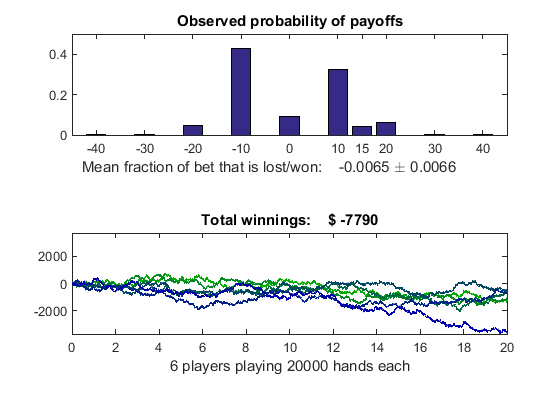# Distributed Blackjack

This example uses the Parallel Computing Toolbox™ to play the card game of blackjack, also known as 21. We simulate a number of players that are independently playing thousands of hands at a time, and display payoff statistics. Simulating the playing of blackjack is representative of Monte Carlo analysis of financial instruments. The simulation can be done completely in parallel, except for the data collection at the end.

For details about the computations, view the code for pctdemo_setup_blackjack.

Related examples:

### Analyze the Sequential Problem

Because the blackjack players are independent one of another, we can simulate them in parallel. We do this by dividing the problem up into a number of smaller tasks.

### Load the Example Settings and the Data

The example uses the default profile when identifying the cluster to use. Discover Clusters and Use Cluster Profiles explains how to create new profiles and how to change the default profile. If you want to use a different example difficulty level or number of tasks, use `paralleldemoconfig` and then run this example again.

```[difficulty, myCluster, numTasks] = pctdemo_helper_getDefaults(); ```

We get the number of players and the number of hands each player plays from `pctdemo_setup_blackjack`. The `difficulty` parameter controls the number of players that we simulate. You can view the code for pctdemo_setup_blackjack for full details.

```[fig, numHands, numPlayers] = pctdemo_setup_blackjack(difficulty); ```

### Divide the Work into Smaller Tasks

We divide the simulation of the `numPlayers` players among the `numTasks` tasks. Thus, task `i` simulates `splitPlayers{i}` players.

```[splitPlayers, numTasks] = pctdemo_helper_split_scalar(numPlayers, ... numTasks); fprintf(['This example will submit a job with %d task(s) ' ... 'to the cluster.\n'], numTasks); ```
```This example will submit a job with 4 task(s) to the cluster. ```

### Create and Submit the Job

We create a job and one task in the job for each split. Notice that the task function is the same function that was used in the sequential example. You can view the code for pctdemo_task_blackjack for full details.

```startTime = clock; job = createJob(myCluster); for i = 1:numTasks createTask(job, @pctdemo_task_blackjack, 1, ... {numHands, splitPlayers(i)}); end ```

We can now submit the job and wait for it to finish.

```submit(job); wait(job); ```

### Retrieve the Results

Let us verify that we received all the results that we expected. `fetchOutputs` will throw an error if the tasks did not complete successfully, in which case we need to delete the job before throwing the error.

```try jobResults = fetchOutputs(job); catch err delete(job); rethrow(err); end ```

Collect the task results into a numHands-by-numPlayers matrix.

```S = cell2mat(jobResults'); ```

We have now finished all the verifications, so we can delete the job.

```delete(job); ```

### Measure the Elapsed Time

The time used for the distributed simulations should be compared against the time it takes to perform the same set of calculations in the Sequential Blackjack example. The elapsed time varies with the underlying hardware and network infrastructure.

```elapsedTime = etime(clock, startTime); fprintf('Elapsed time is %2.1f seconds\n', elapsedTime); ```
```Elapsed time is 27.5 seconds ```

### Plot the Results

We display the expected fraction of the bet that is won or lost in each hand, along with the confidence interval. We also show the evolution of the winnings and losses of each of the players we simulate. You can view the code for pctdemo_plot_blackjack for full details.

```pctdemo_plot_blackjack(fig, S); ```## SupportGet trial now